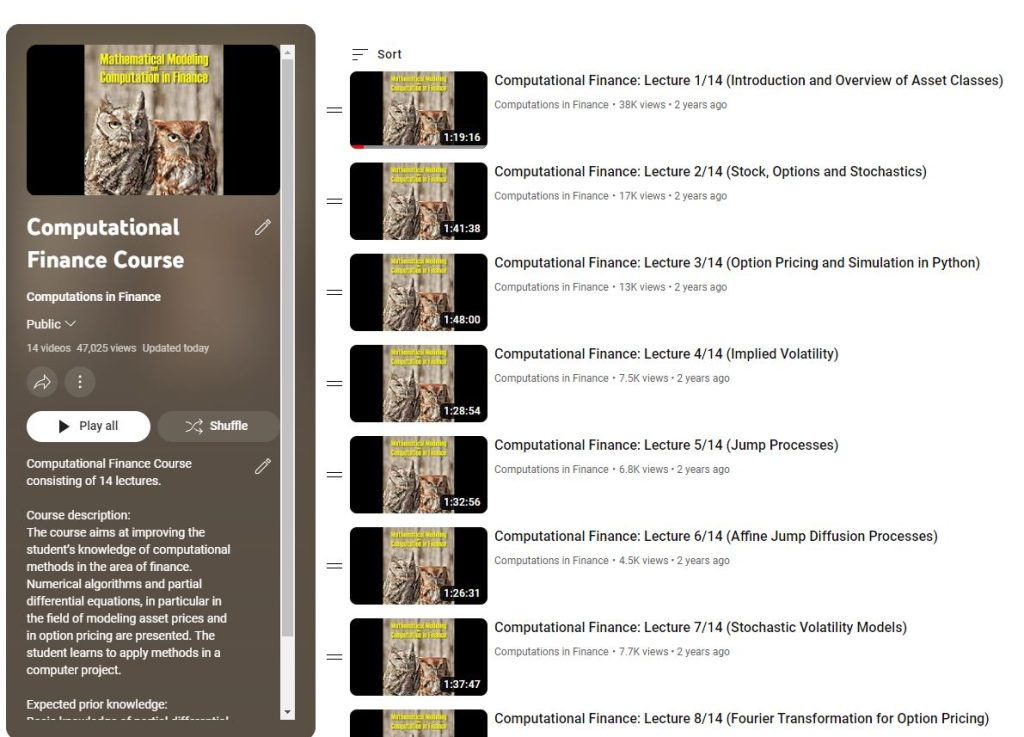# Computational Finance

Course Description:
The course aims to improve the student’s knowledge of computational methods in finance. Numerical algorithms and partial differential equations, in particular in the field of modelling asset prices and in option pricing, are presented. The student learns to apply methods in a computer project.

Expected Prior Knowledge:
Basic knowledge of partial differential equations (PDEs), numerical methods for solving PDEs, of linear algebra and of the basic of numerical linear algebra, computing tool: Python.

Course Materials:

1. Slides can be found here.
2. The book used in the course can be get here.
3. Codes and the exercises for the book can be found here.
4. Youtube Channel with courses can be found here.### Lecture 1- Introduction and Overview of Asset Classes

1.1. Introduction
1.2. *Background Knowledge, Materials for the course (book etc.)
1.3. *Outline of the Course
1.5. Financial Engineering
1.6. Financial Markets and Different Asset Classes
1.7. Stocks and Dividends
1.8. Interest Rates
1.9. Volatility
1.10. Options & Payoffs

### Lecture 2- Stock, Options and Stochastics

2.1. Trading of Options and Hedging
2.2. Commodities
2.3. Currencies and Cryptos
2.4. Value of Call and Put Options and Hedging
2.5. Modeling of Asset Prices and Randomness
2.6. Stochastic Processes for Stock Prices
2.7. Ito’s Lemma for Solving SDEs

### Lecture 3- Option Pricing and Simulation in Python

3.1. Stock Paths and Simulation in Python
3.2. Black-Scholes model
3.3. Hedging with the Black-Scholes model
3.4. Martingales and Option Pricing
3.5. Coding of Martingales in Python
3.6. Risk Neutral Valuation and Feynman-Kac Formula
3.7. Measures and Impact on a Drift
3.8. Closed-Form Solution for Black-Scholes model

### Lecture 1- Introduction and Overview of Asset Classes

1.1. Introduction
1.2. *Background Knowledge, Materials for the course (book etc.)
1.3. *Outline of the Course
1.5. Financial Engineering
1.6. Financial Markets and Different Asset Classes
1.7. Stocks and Dividends
1.8. Interest Rates
1.9. Volatility
1.10. Options & Payoffs

### Lecture 4- Implied Volatility

4.1. Key Elements for Pricing Derivatives
4.2. Black-Scholes Implied Volatility
4.3. Newton-Raphson Method and Implementation in Python
4.4. Time-Dependent Volatility Parameter, σ(t)
4.5. Implied Volatility Surface
4.6. Deficiencies of the Black-Scholes Model

### Lecture 5- Jump Processes

5.1. Inclusion of Jumps in the Stock Process
5.2. Poisson Process and Implementation in Python
5.3. Itˆo’s Lemma and Jumps
5.4. Jumps and Asset Dynamics under the Q-Measure
5.5. Partial Integro-Differential Equations
5.6. Different Jump Distributions and Implied Volatility
5.7. Expectation and Jump Processes
5.8. Characteristic Function for a Jump Process
1.10. Options & Payoffs

### Lecture 6- Affine Jump Diffusion Processes

6.1. How to Choose a Pricing Method?
6.2. Fourier Transformation- Motivation
6.3. Characteristic Function for the Black-Scholes Model
6.4. Affine Diffusion Processes
6.5. Characteristic Function for High Dimensions
6.6. Affine Jump Diffusion Processes

### Lecture 7- Stochastic Volatility Models

7.1. Towards Stochastic Volatility
7.2. The Stochastic Volatility Model of Heston
7.3. Correlated Stochastic Differential Equations
7.4. Ito’s Lemma for Vector Processes
7.5. Pricing PDE for the Heston Model
7.6. Impact of SV Model Parameters on Implied Volatility
7.7. Black-Scholes vs. Heston Model
7.8. Characteristic Function for the Heston Model

### Lecture 8- Fourier Transformation for Option Pricing

8.1. Fourier Transformation
8.2. FFT- Fast Fourier Transformation in Python
8.3. The COS Method and Density Recovery
8.4. Implementation of the COS Method in Python
8.5. European Option Pricing with Characteristic Function
8.6. Pricing Experiments Using COS Method in Python

### Lecture 9- Monte Carlo Simulation

9.1. Monte Carlo and Integration via Sampling
9.2. Examples of Stochastic Integrals in Python
9.3. Smoothness of a Payoff and Impact on Convergence
9.4. Types of Convergence
9.5. Option Pricing and Standard Error
9.6. Euler Discretization
9.7. Milstein Discretization

### Lecture 10- Monte Carlo Simulation of the Heston Model

10.1. Option Pricing with Monte Carlo
10.2. Simulation of the CIR Process
10.3. Exact Simulation of the CIR Model
10.4. Almost Exact Simulation of the Heston Model
10.5. The Heston Model and Simulation in Python

### Lecture 11- Hedging and Monte Carlo Greeks

11.1. Hedging with the Black-Scholes Model
11.2. Dynamic Hedging- Python Experiment
11.3. Hedging with Jumps
11.4. Delta, Gamma and Vega Hedging
11.5. Monte Carlo Sensitivity: Finite Difference
11.6. Monte Carlo Sensitivity: Pathwise Sensitivities
11.7. Monte Carlo Sensitivity: Likelihood Ratio Method

### Lecture 12- Forward Start Options and Model of Bates

12.1. Forward-Start Options
12.2. Characteristic Function for Pricing of Forward Start Options
12.3. Forward Start Options under the Black-Scholes Model
12.4. Forward Start Options under the Heston Model
12.5. Forward Implied Volatility with Python
12.6. The Bates Model
12.7. Variance Swaps

### Lecture 13- Exotic Derivatives

13.1. Overview of Payoffs in the Industry
13.2. Binaries and Digitals
13.3. Path-Dependent Options: Barrier Options
13.4. Asian Options
13.5. Multi-Asset Options

### Lecture 14- Summary of the Course

14.1. Summary of the Course